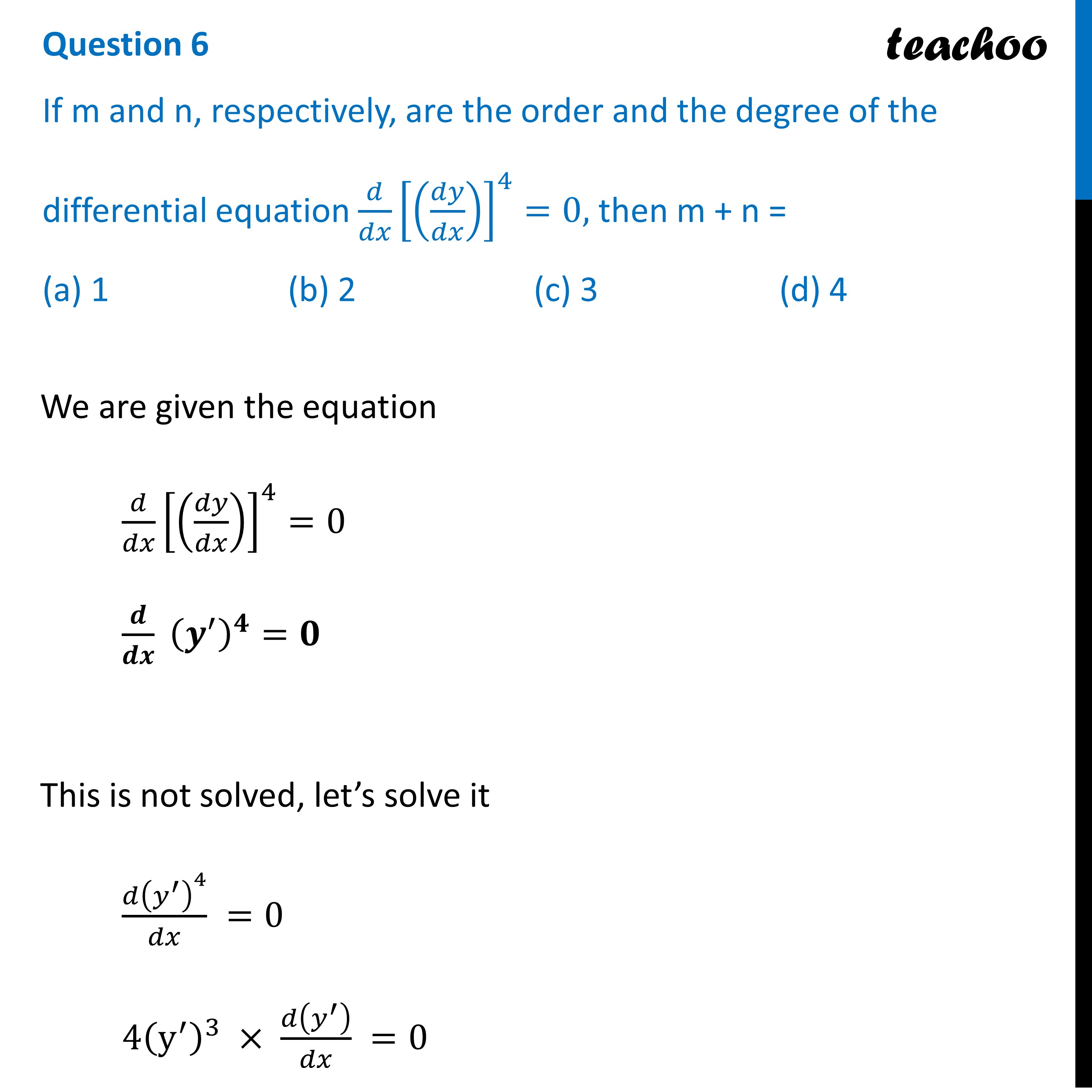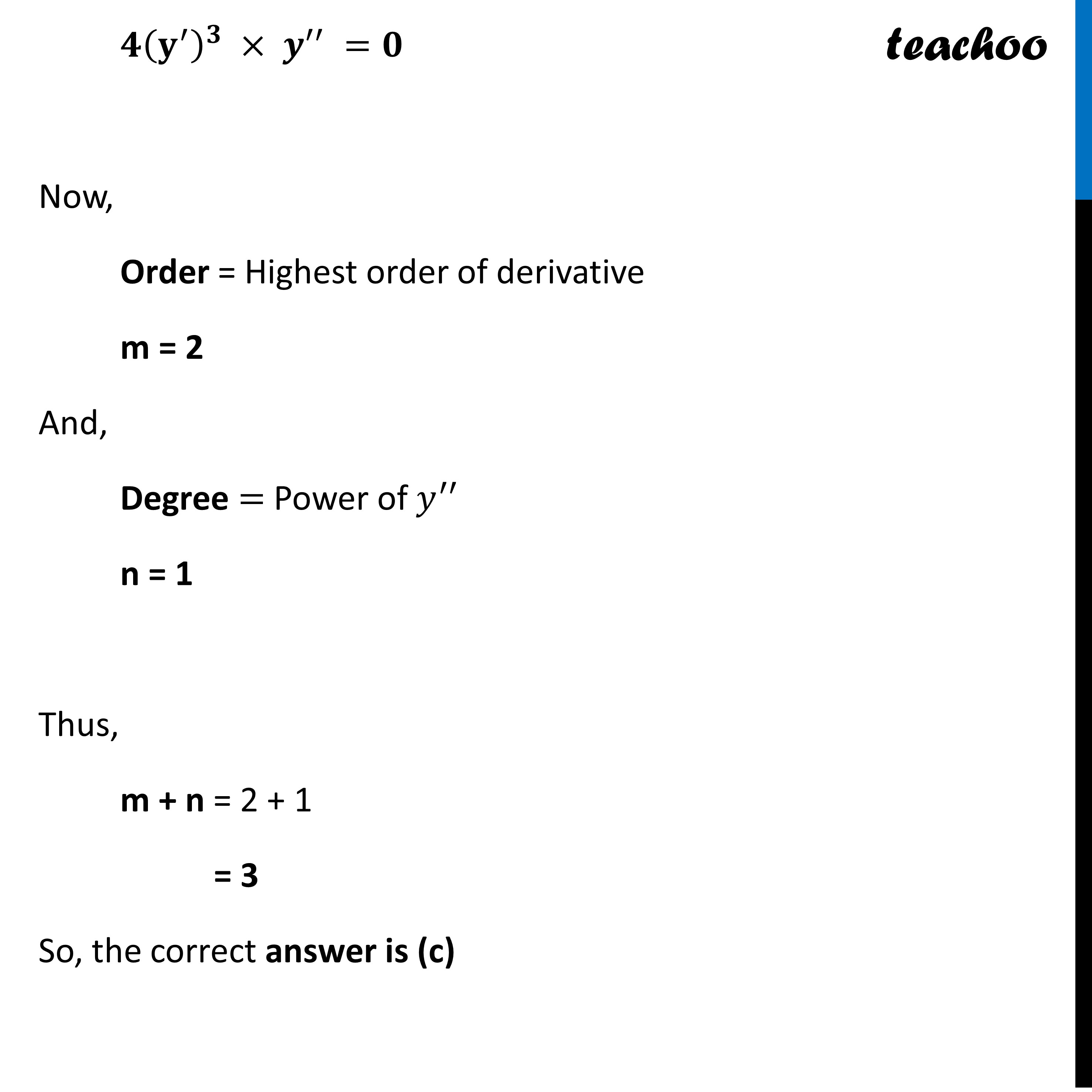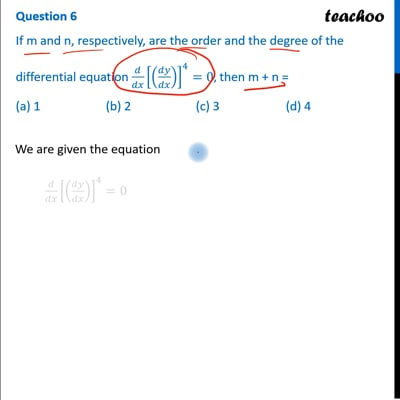CBSE Class 12 Sample Paper for 2023 Boards

Class 12
Solutions of Sample Papers and Past Year Papers - for Class 12 Boards

## (d) 4

This question is Similar to Question - 2 CBSE-Class-12-Sample-Paper-for-2022-Boards-For-Term-2This video is only available for Teachoo black users

Get live Maths 1-on-1 Classs - Class 6 to 12

### Transcript

Question 6 If m and n, respectively, are the order and the degree of the differential equation 𝑑/𝑑𝑥 [(𝑑𝑦/𝑑𝑥)]^4=0, then m + n = (a) 1 (b) 2 (c) 3 (d) 4 We are given the equation 𝑑/𝑑𝑥 [(𝑑𝑦/𝑑𝑥)]^4=0 𝒅/𝒅𝒙 (𝒚^′ )^𝟒=𝟎 This is not solved, let’s solve it (𝑑(𝑦^′ )^4)/𝑑𝑥 =0 4(y^′ )^3 × 𝑑(𝑦^′ )/𝑑𝑥 =0 𝟒(𝐲^′ )^𝟑 × 𝒚^′′ =𝟎 Now, Order = Highest order of derivative m = 2 And, Degree = Power of 𝑦^′′ n = 1 Thus, m + n = 2 + 1 = 3 So, the correct answer is (c)